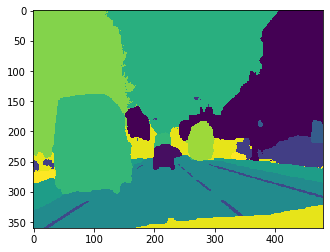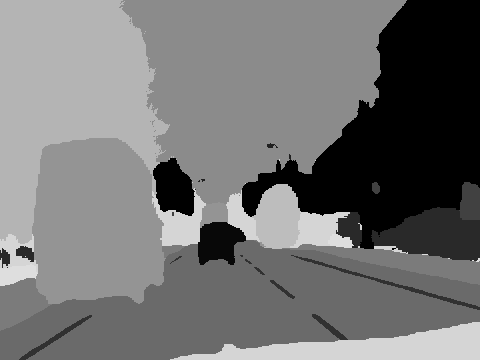# Saving Segmentation Masks - Tutorial

Hi all,

While working through my material one thing I decided to show was how to save our made segmentation masks into `.png`'s, similar to how CamVid’s are. Here is how we do so (this works for both fastai1 and 2):

First, we’ll grab some predictions:
`preds = learn.get_preds()`
From here, if we check out the shape we get the following:

``````preds.shape
torch.Size([5, 32, 360, 480])
``````

What does this mean? My batch that I sent in had 5 images, so they are all stacked in a row. From here, we can then grab the actual classes by doing the following:

``````pred_1 = preds
pred_argmax = pred_1.argmax(dim=0)
``````

Now we get the following if we do `plt.imshow(pred_argmax)`:Great! So how do I save this away? Like so:

``````pred_argmax = pred_argmax.numpy()
rescaled = (255.0/pred_argmax.max() * (pred_argmax - pred_argmax.min())).astype(np.uint8)
im = Image.fromarray(rescaled)
``````

Now our image (if we do `im`) looks like so:All that’s left is to save it away!

`im.save('test.img')`

1 Like

I recently had to deal with something similar. As I needed to visualize and calculate metrics many times after training, predicting it was taking too long. The difference is I stored the tensor directly with torch.save in order to not loose any value due to compression, but for just visualizing saving as image would indeed save quite a bit of space ^^.

1 Like

Which do you think is better in production? (I was helping someone for doing this/figuring it out)

I’m sure with the right parameters you could save image in lossless format too, it just required less of my time to save the tensor than to figure out how that worked. I would say it depends on what will you use it for. In the common situation of serving a model to a user, giving back the image is fine, but what if you gave back tensor of probabilities and allowed the user to change the threshold in real time and visualize result (thinking of binary case here)? It also allows showing the probability map which is quite interesting in some cases. I am doing a comic text detector and while when I use threshold I can see the text, when I show the probability map I can see that while the text has the highest value, all the area around it (speech bubble) has higher value than background too.

1 Like

Assuming you only want to save the final mask, it would be fine to save as .png as it uses lossless compression.

If you want to keep all the original predictions and compress to save disk space, one option would be to save and compress the tensors themselves, or convert to numpy first. This Stack Overflow question has multiple ideas, although some might be out of date.

2 Likes

Hello, I am currently trying to add a class in the camvid dataset, and then train the entire model on 33 classes.

For that I have saved a trained CAMVID model and I am using that to make predictions on my dataset. I add my additional mask in the image so that the number of classes increases to 33. Using this same approach.

``````for i, name in tqdm(zip(range(len(pred12)), fnames[:100])):
tensorr = pred12[i].argmax(dim=0).numpy()
index = int(name.name[:-4].split('_'))
print(index)

rescaled = (255 / new.max() * (new - new.min())).astype(np.uint8)
# print(rescaled)

im = Image.fromarray(rescaled, 'L')
# plt.imshow(im, 'Greys')
# im.save('test2-L.png')

print(new)
im_new = Image.fromarray(new, 'L') # 'L' for 8 bit black and white image
# plt.imshow(rescaled, 'Greys')
im_new.save('new-L.png')
# im.save(f'/content/proper/{name.name[:-4]}_P.png')
break
``````

However, when I try to merge these 2 datasets (CAMVID + my dataset), the number of classes goes up to 57. (Because after rescaling the numbers are between 0 and 255) Is there a way to save the images, so that the saved images have their values in the range between 0 - 32? Saving the rescaled images does not fix this issue. I tried different ways to save the image, but none to them seem to work. Any help would be appreciated